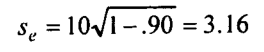# Standard Error of Measurement

The reliability of a test may be expressed in terms of the standard error of measurement (se), also called the standard error of a score. This score is particularly well suited to the interpretation of individual scores. For many testing purposes, it is, therefore, more useful than the reliability coefficient. The standard error of measurement can be easily computed from the reliability coefficient of the test by a simple rearrangement of the Rulon formula (g):in which s, is the standard deviation of the test scores and rtt the reliability coefficient, both computed on the same group.

The standard error of measurement is a useful statistic because it enables an experimenter to estimate limits within which the true scores of a certain percentage of individuals (subjects) having a given observed score can be expected to fall, assuming that errors of measurement are normally distributed.

According to the normal law of probability, about 68% (more precisely 68.27%) of a group of individuals will have true scores falling within ±1 standard error of the measurement of the observed score.

Likewise, about 95% of a group of individuals having the same observed scores will have true scores falling within ±2 standard error of the measurement of the observed score.

And virtually all (99.72%) will have true scores falling within+3 standard error of the measurement of that observed score.

As an example, assume that the standard deviation of the observed scores on a test is 10 and the reliability coefficient is 0.90; then;So if a person’s observed score is 50, it can be concluded with 68.27% confidence that this person’s true score lies in the interval 50 ±1(3.16), i.e. between 46.84 and 53,16. If we want to be more certain of our prediction, we can choose higher odds than the above.

Thus we are virtually certain (99.72%) that this individual will have his true score in the range 40.52 and 59.48 resulting from the interval 50±3(3.I6).

As formula (k) shows, the standard error of measurement increases as reliability decreases. When rn=1.0, there is no error at all in estimating an individual’s true score from the observed score.

When rn=.00, the error of measurement is a maximum and equal to the standard deviation of the observed score.

Of course, a test with a reliability coefficient close to 0.00 is useless because the correctness of any decisions made on the basis of the scores will be no better than chance.

How do we judge the usefulness of a test in terms of the reliability coefficient

The answer to this question depends on what one plans to do with the test score.

If a test is used to determine whether the mean scores of two groups of people are significantly different, then a reliability coefficient as low as 0.65 may be satisfactory. If the test is used to compare one individual with another, a coefficient of at least 0.85 is needed.

More 'Reliability and Validity in Measurement' Posts ⁄
Related Posts ⁄